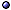Bianchi Classification of Cosmological Models

In General > s.a. Homogeneity; Isotropic Metric.
* Idea: It is a classification of most spatially homogeneous anisotropic cosmologies; What Bianchi really did was to classify the three-dimensional Lie algebras, but the Lie algebra can be taken to be that of the isometry group, and the two things become equivalent; The original scheme has been later modified.
\$ Def: Spacetimes with a 3D group G of isometries acting freely and (simply) transitively on spacelike hypersurfaces.
* Motivation: The vacuum or perfect fluid models give simple equations, which however are non-linear enough to study anisotropy, singularities, chaotic dynamics, horizon structure, gravitational waves, non-Newtonian Weyl curvature, etc.
* Classification: If the Lie algebra is generated by Killing vector fields ξi (i = 1, 2, 3), with commutators [ξi, ξj] = Cijk ξk, choose a triad of left-invariant vector fields Li such that

Cijk = εijl nlk + δjk viδik vj ,

where nij = diag(n1, n2, n3), ni = ±1, 0, and vi = (0,1; 0,1; 0,1), with vi nij = 0; Types are classified by the combinations of values of ni and vi (class A has vi = 0, class B has vi ≠ 0); The dual forms χi are also left-invariant.
* Killing vector fields: They generate the isometries, so they are right-invariant and satisfy

[ξi, ξj]a = Ckij ξka ,   [ξ, η]a = Cabc ξb ηc .

* Left-invariant forms: There are three 1-forms $$\sigma^i{}_a$$, such that qab = gij σia σ jb and ∇[a σib] = −$$1\over2$$Ccab σic.
* Metric: It is often parametrized in an ADM-like form by a volume element and a shear matrix β, such that

ds2 = −N2(t) dt2 + gij(t) σiσj ,   gij = exp{−2Ω(t)} (exp{2β(t)})ij ;

the simplest case is for types I, II, VIII, IX, in which g or β are diagonalizable; With some loss of generality,

gij = e−2Ω(t) diag(a(t), b(t), 1/ab) ,   βij = diag(β+ + 31/2 β, β+ − 31/2 β, − 2β+) .

Special Cases and Models > s.a. bianchi I, bianchi IX, and other models; scalar-tensor theories.
@ Class / type A: Schücking et al GRG(03) [diagram]; Apostolopoulos CQG(03)gq [self-similar]; Ferragut et al JGP(12) [with k = 1, analytic integrability].
@ Class / type B: Apostolopoulos CQG(05)gq/04 [self-similar]; Yamamoto PRD(12)-a1108 [with electromagnetic fields].
@ Compact: Koike et al JMP(94) [topologies]; Tanimoto et al JMP(97)gq/96, JMP(97)gq; Coley & Goliath PRD(00)gq [fluid + scalar]; Kodama PTP(02)gq/01 [fluid]; Torre CQG(04)gq [weakly locally homogeneous].Specific models: see bianchi I models; bianchi II-V models; bianchi VI-VIII models; bianchi IX models.

References > s.a. geodesics; minisuperspace quantum cosmology; neural networks; quasilocal energy; types of singularities.
@ Early references: Bianchi MMSI(1897), translation GRG(01); Bianchi 18; Misner PR(69) [ADM].
@ Reviews: in Misner et al 73; MacCallum in(73)-a2001; Ryan & Shepley 75; MacCallum in(79); Jantzen in(84); Ellis GRG(06).
@ EMW variables: Ellis & MacCallum CMP(69); MacCallum & Ellis CMP(70); MacCallum CMP(71); Wainwright in(88); Wainwright & Hsu CQG(89); Wainwright & Ma in(92); Hewitt & Wainwright CQG(93).
@ Ashtekar variables: Kodama PTP(88); Ashtekar & Pullin in(90); Ashtekar & Samuel CQG(91); Manojlović & Miković CQG(93); Obregón et al PRD(93)gq; González & Tate CQG(95)gq/94 [I and II]; Calzetta & Thibeault gq/97/CQG [I, II, IX].
@ Symmetries and reduction: Coussaert & Henneaux CQG(93)gq; Schirmer CQG(95); Capozziello et al IJMPD(97) [Noether symmetries and general relativity]; Christodoulakis et al CMP(02)gq/01 [invariant characterization]; Apostolopoulos CQG(05) [new approach].
@ Hamiltonian / Lagrangian: Ryan & Waller gq/97 [class B]; Tanimoto et al JMP(97)gq [compact]; Pons & Shepley PRD(98)gq.
@ Dynamics, general: Bonilla CQG(98); Byland & Scialom PRD(98)gq [I, II, Kantowski-Sachs]; Billyard PhD(99)gq; Szydłowski & Krawiec IJMPA(00) [constraint solved]; Aguiar & Crawford PRD(00)gq [I + III, dust and Λ]; Barrow & Hervik CQG(02)gq [Weyl curvature invariant]; Gambini & Pullin CQG(03) [discretized]; Fay gq/05-in [+ massive scalar, ADM]; Goheer et al CQG(07)-a0710 [in f(R) theories].
@ Stability: Barrow & Sonoda PRP(86) [several V, VI, VII models]; Zotov gq/99.
@ Isotropization: Cervantes-Cota & Chauvet PRD(99)gq/98 [I-V-IX, induced gravity]; Fay CQG(03) [class A], gq/05-in [scalar-tensor]; Barzegar et al a1904 [massless Einstein-Vlasov system]; > s.a. types I, VII, IX.
@ In Einstein-Yang-Mills theory: Donets et al PRD(99) [N = 2 supersymmetric].
@ In higher-order theories: Querella PhD(98)gq/99; Barrow & Hervik PRD(06)gq [I + II, quadratic theories]; Middleton CQG(10) [I, VIII, IX, anisotropy and approach to singularity].
@ Other theories: Savaria gq/97 [non-symmetric gravity]; Bonneau CQG(98) [Einstein-Weyl spaces]; Cognola & Zerbini IJTP(08)-a0802 [generalized gravity theories]; > s.a. modified electrodynamics; non-commutative physics.
@ Kinds of matter: Rendall & Uggla CQG(00)gq [Einstein-Vlasov]; Calogero & Heinzle PhyD(11)-a0911 [anisotropic matter]; Normann et al CQG(18)-a1712 [p-form gauge fields]; Thorsrud CQG(19)-a1905 [free massless scalar field].
@ Phenomenology: Coley & Lim CQG(07) [bounds on shear]; > s.a. cmb anisotropy.
@ Related topics: Estabrook et al JMP(68); Siklos CMP(78) [horizons and whimper singularities]; Rosquist & Jantzen PRP(88); Fujiwara et al CQG(93)gq; Di Pietro & Demaret IJMPD(99)gq [duality]; De Ritis et al NCB(01) [horizons]; Doliwa et al JGP(04) [discretization]; Hervik ap/05, Palle ap/05 [re vorticity, > s.a. cmb]; Heinzle & Uggla CQG(10) [monotonic functions]; Avetisyan & Verch CQG(13)-a1212 [harmonic and spectral analysis].